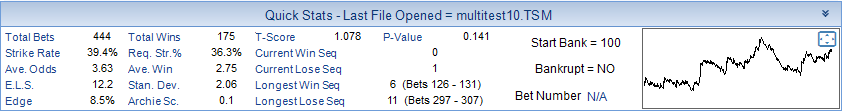# T-Score and P-Value

A few years ago we added the Archie Score into the TSM Quick Stats. Based on the Chi Square Formula it attempts to tell us the probability that a set of results is down to chance.

Recently the T-Score and the linked P-Value have been added into the TSM Quick Stats.

This is another way of looking at whether the results are down to chance or genuine skill. T-Score is only valid for positive yield. For Level Stakes Betting the yield is the same as your ROI or Edge at Level Stakes.

The Calculations used in TSM are based on the Excel Calculator provided by Joseph Buchdahl at Football-Data.co.uk. A download of the Excel Calculator can be found on the linked web page. For that reason I will not be explaining the exact calculations. I will simply summarise the T-Score and P-Value below.

In short, a higher T-Score translates to a higher likelihood of the yield not being the result of chance. In other words, the higher the T-Score the better.

The P-Value is the T-Score converted to probability. A higher T-Score leads to a lower P-Value.

P-Value can range from 0.5 – 0.

The P-Value is the likelihood that the yield of the betting data is the result of chance.

In general you can consider a P-Value below 0.05 or 5% as giving moderate evidence that the yield is NOT as a result of chance alone.

A P-Value below 0.01 or 1% could be considered strong evidence that the yield is NOT as a result of chance alone.

In the TSM V7 Desktop Screenshot below we can see the Quick Stats from a decent sample of bets.

We can see an Archie Score of 0.1. Straight away this suggests a high chance of the results being down to chance. We then look at the P-Value is above the 0.05 threshold (5%) which means we cannot say with any confidence that the results our down to skill. As the number of bets increase from 444 to a larger sample size we can expect the T-Score to increase and the linked P-Value to decrease.The T-Score and P-Value are included in both the TSM Desktop (Windows) and the TSMapp (Browser Version).

When using TSM, If you hover your mouse over either the T-Score and P-Value Labels in TSM, a tool tip reminder pops up explaining what each one is and what values we are looking for.